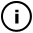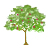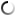# Sunday, January 24, 2010

## Brownian motion in polar coordinates

int frame = 0;

float distance = 0, distance2 =0,angle = 0,angle2 = 0;

void setup() {
size(800,600);
background(255);
smooth();
fill(255,0,0,10);
noStroke();
}

void draw() {
frame++;
//background(255,255,255,10);
// Draw points
for( int i = 0; i < 100; i++ ) {
distance = distance2;
angle = angle2;

int x = int(distance * cos(angle));
int y = int(distance * sin(angle));

distance2 = distance+random(-0.9,0.9);
angle2 = angle+random(-0.1,0.1);
int x2 = int( distance2* cos(angle2));
int y2 = int(distance2 * sin(angle2));

//noStroke();
//ellipse(width/2+x,height/2+y,4,4);

stroke(104, y/2, x/2,40);
strokeWeight(1);     line(width/2+x2*1.2,height/2+y2*1.2,width/2+x*1.2,height/2+y*1.2);
}

}

float randomNonNormal()
{
float x = 1.0, y = 1.0,
s = 2.0; // s = x^2 + y^2
while(s >= 1.0)
{
x = random(-1.0f, 1.0f);
y = random(-1.0f, 1.0f);
s = x*x + y*y;
}
return x * sqrt(-15.0f * log(s)/s);
}

## infosubmitted by: DARYL_Gamma
views:

Based on the "furry ball" sketchThis sketch has a parent
Tags:

## commentsloading...